# Euler angles

(diff) ← Older revision | Latest revision (diff) | Newer revision → (diff)

The angles,andthat determine the position of one Cartesian rectangular coordinate system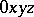relative to another one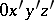with the same origin and orientation. The Euler angles are regarded as the angles through which the former must be successively rotated about the axes of the latter so that in the end the two systems coincide (see Fig.).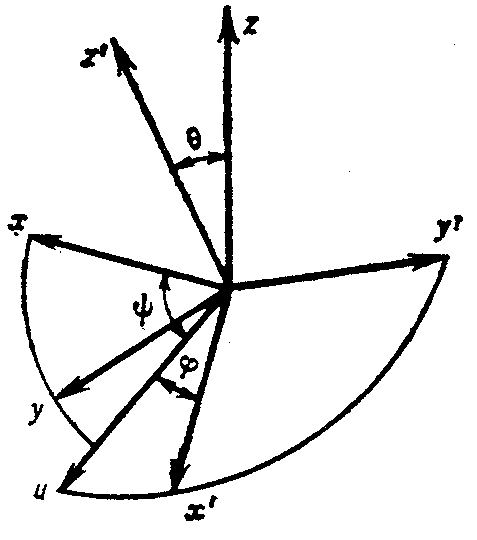Figure: e036390a

Letbe the axis coinciding with the line of intersection of the planes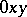and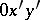, oriented so that the three lines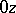,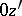andform a right-handed triple. Thenis the angle between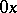and, measured in the planefromin the direction of the shortest rotation ofto,is the angle betweenandnot exceeding, andis in the direction of the shortest rotation ofto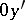. The coordinates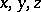, andare connected by the relations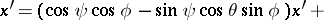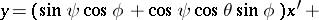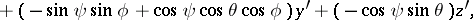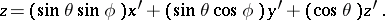These angles were introduced by L. Euler (1748).